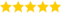# How to Use IF ELSE Statements in Python?

Last updated on 13th Oct 2020, Artciles, Blog

Akash (Sr Technical Project Manager )

High level Domain Expert in TOP MNCs with 8+ Years of Experience. Also, Handled Around 16+ Projects and Shared his Knowledge by Writing these Blogs for us.(5.0) | 12547 Ratings 2165

### What is an if…else statement in Python?

• Decision making is required when we want to execute a code only if a certain condition is satisfied.
• The if…elif…else statement is used in Python for decision making.

### Python if Statement Syntax

if test expression:

statement(s)

• Here, the program evaluates the test expression and will execute statement(s) only if the test expression is True.
• If the test expression is False, the statement(s) is not executed.
• In Python, the body of the if statement is indicated by the indentation. The body starts with an indentation and the first unindented line marks the end.
• Python interprets non-zero values as True. None and 0 are interpreted as False.

### Example: Python if Statement

# If the number is positive, we print an appropriate message

• num = 3
• if num > 0:
•     print(num, “is a positive number.”)
• print(“This is always printed.”)
• num = -1
• if num > 0:
•     print(num, “is a positive number.”)
• print(“This is also always printed.”)

### Output:

3 is a positive number

This is always printed

This is also always printed.

• In the above example, num > 0 is the test expression.
• The body of if is executed only if this evaluates to True.
• When the variable num is equal to 3, test expression is true and statements inside the body of if are executed.
• If the variable num is equal to -1, test expression is false and statements inside the body of if are skipped.
• The print() statement falls outside of the if block (unindented). Hence, it is executed regardless of the test expression.

### Syntax of if…else

• if test expression:
•     Body of if
• else:
•     Body of else
• The if..else statement evaluates test expression and will execute the body of if only when the test condition is True.
• If the condition is False, the body of else is executed. Indentation is used to separate the blocks.

### Example : if…else

• # Program checks if the number is positive or negative
• # And displays an appropriate message
• num = 3
• # Try these two variations as well.
• # num = -5
• # num = 0
• if num >= 0:
•     print(“Positive or Zero”)
• else:
•     print(“Negative number”)

### Output

Positive or Zero

• In the above example, when num is equal to 3, the test expression is true and the body of if is executed and the body of else is skipped.
• If num is equal to -5, the test expression is false and the body of else is executed and the body of if is skipped.
• If num is equal to 0, the test expression is true and body of if is executed and body of else is skipped.

### Syntax of if…elif…else

• if test expression:
•     Body of if
• elif test expression:
•     Body of elif
• else:
•     Body of else
• The elif is short for else if. It allows us to check for multiple expressions.
• If the condition for if is False, it checks the condition of the next elif block and so on.
• If all the conditions are False, the body of else is executed.
• Only one block among the several if…elif…else blocks is executed according to the condition.
• The if block can have only one else block. But it can have multiple elif blocks.

### Example : if…elif…else

”’In this program,

we check if the number is positive or

negative or zero and

display an appropriate message”’

• num = 3.4
• # Try these two variations as well:
• # num = 0
• # num = -4.5
• if num > 0:
•     print(“Positive number”)
• elif num == 0:
•     print(“Zero”)
• else:
•     print(“Negative number”)
• When the variable num is positive, Positive number is printed.
• If num is equal to 0, Zero is printed.
• If num is negative, Negative number is printed.

### Python Nested if statements

• We can have a if…elif…else statement inside another if…elif…else statement. This is called nesting in computer programming.
• Any number of these statements can be nested inside one another. Indentation is the only way to figure out the level of nesting. They can get confusing, so they must be avoided unless necessary.

### Python Nested if Example

”’In this program, we input a number

check if the number is positive or

negative or zero and display

an appropriate message

This time we use nested if statement”’

• num = float(input(“Enter a number: “))
• if num >= 0:
•     if num == 0:
•         print(“Zero”)
•     else:
•         print(“Positive number”)
• else:
•     print(“Negative number”)

### Output 1

Enter a number: 5

Positive number

### Output 2

Enter a number: -1

Negative number

### Output 3

Enter a number: 0

Zero

Are you looking training with Right Jobs?